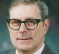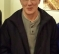## Delay estimation using cross correlationStarted by 7 months ago6 replieslatest reply 7 months ago371 views

Hi

Suppose there are two continuous signals of same frequency say 4 KHz. The delay/time corresponding to its one cycle is around 250 us. If we delay one signal by 4010 us (i.e >> one cycle delay), can we use cross correlation techniques to estimate this delay accurately?

Thanks

[ - ]The signal characteristics matter a lot.  This sort of thing is done in practice a lot but the signal must have sufficient entropy and auto-correlation properties to make it work.  Pseudo-random sequences or things like Barker codes or sequences with a sharp auto-correlation peak are typically used for this application.  If the pulses are filtered sharply, for example with a Raised-Cosine filter with very low excess bandwidth, then oversampling the symbols allows time synchronization to a small fraction of a symbol.

Naturally the other side of this is that if you don't have control of the signal to put such features in it, then it can be extremely difficult to get good time delay estimates if the signal properties don't support it.

[ - ]I don't understand what this has to do with DSP (Digital Signal Processing). You can't have continuous signals in DSP. They must be sampled and, usually, quantized.

So let's drop the term continuous.

Next you say the signals are of the same frequency. "Frequency" is singular. So the two signals are sinusoids. Since you say that the period of each signal is "around" 250 microseconds, you don't know the exact frequency, only that the two are the same frequency. The reciprocal of ~250 microseconds is ~4000 Hz.

The exact cross-correlation function of the two signals (continuous) is a sinewave and it has all positive peaks at the relative delay, the peaks equally spaced by multiples of the period.

If you have sampled the two signals, you undoubtedly have some finite length. If that available length is large compared to the period, you will still have equally spaced peaks at the relative delay, but they will no longer be equal because the cross-correlation function is multiplied by a triangular window. You have the additional difficulty that the cross-correlation function of two discrete time signals is, itself, sampled. So if, for example, you have 4KHz signals, the true delay is 250 microseconds but if you sample the signals at, say 10KHz, you only get samples of the cross-correlation at multiples of 100 microseconds. So the peaks of the sampled autocorrelation function are not going to all lie on exact multiples of the true delay. In the specific case of 4KH and 10KH, you will get every second peak landing exactly at a sample, but that's only true because the sampling rate is a rational number with a small denominator.

However, if the duration of the sampled signals is quite long, you can increase the sampling rate by interpolation and therefore get improved accuracy.

Let's back off the cross correlation question.  If one of the signals is

A sin (a t + r)

and the other is

B sin (b t + s)

Then after sampling replace t by nT to get all the sample values. Each sample of each of the two waves gives you an equation like w = A sin a n T + r, where w,n, and T are known and A,a, and r are unknown.  A few such equations allows you to solve for A,a, and r. Similarly a few such equations for the other wave allows you to solve for B,b, and s.  Then r-s is your period, exactly.

[ - ]If the delay is more than 3/4 th of cycle (the case given here) there will be ambicuity due to the periodicity of the cosine wave.So we can not estimate the delay.

[ - ]Unless the envelope of the signal changes significantly over the obersvation period, there is no way.

as soon as the signal is broad-band (containing more than a single frequency), the number of possible time-shifts that fulfil all phase shifts between the two signals decreases. If the signal is broadband enough, only one possible time shift remains.

By the way: The envelope of the signal changing significantly is just another way of saying it has to be broadband. A changing envelope is a modulation of the carrier with the envelope spreading the frequency of the carrier the bandwidth of the envelope modulation

[ - ]for digital case you can get accuracy if delay is integer multiples of sample time.

For fractional delay accuracy you need upsampling for that target.

[ - ]